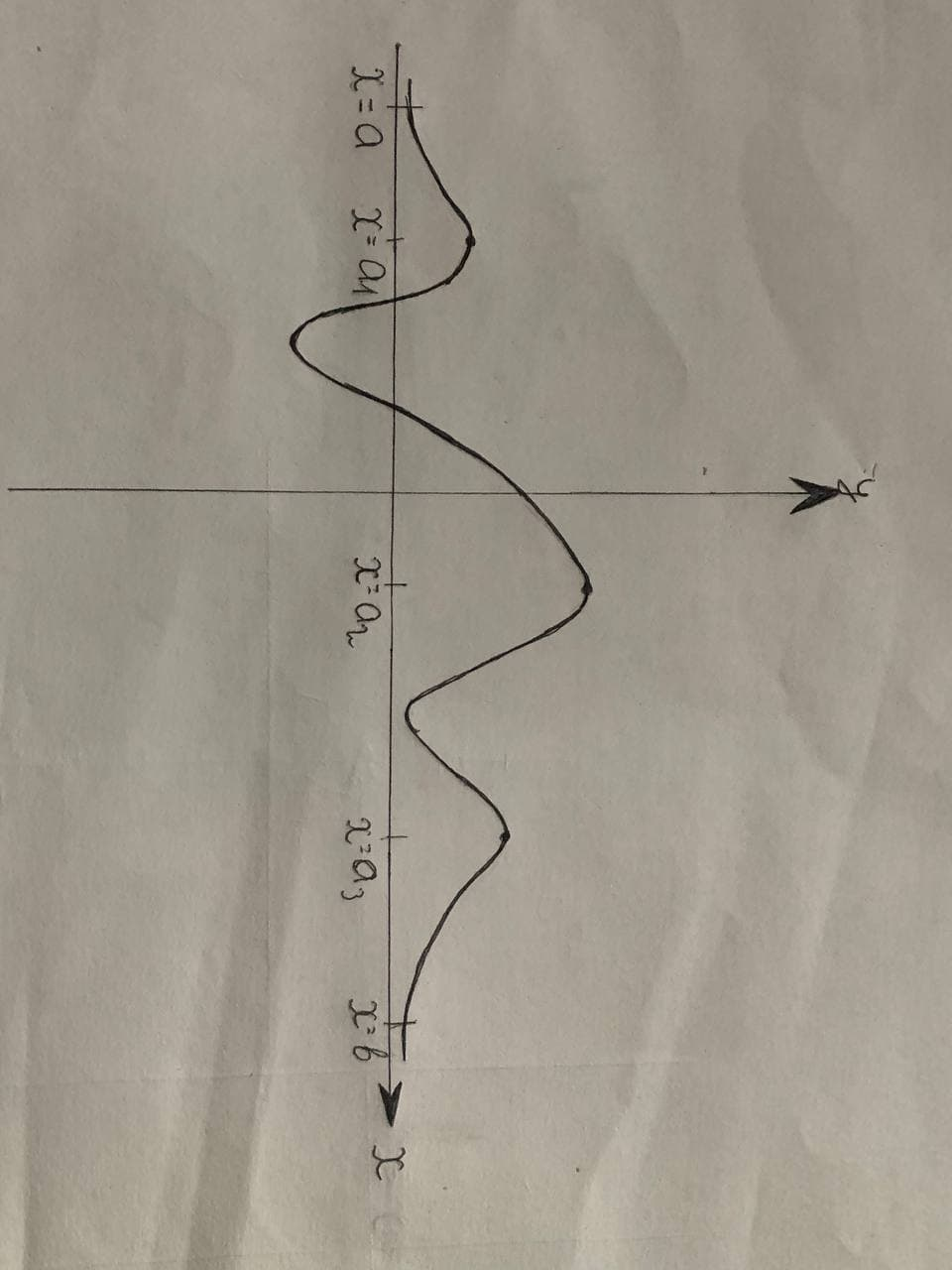What is the difference between a relative maximum and anMahagnazk 2021-11-21 Answered
What is the difference between a relative maximum and an absolute maximum on an interval |?

• Questions are typically answered in as fast as 30 minutes

Solve your problem for the price of one coffee

• Math expert for every subject
• Pay only if we can solve itGladys Fasching

Step 1
Suppose a function given by $$\displaystyle{y}={f}{\left({x}\right)}$$ is defined in the interval (a,b). In order to find the maximum or minimum. value of the function, we make use of the derivative $$\displaystyle{\frac{{{\left.{d}{y}\right.}}}{{{\left.{d}{x}\right.}}}}$$ as it gives the rate of change of quantity ywith respect to quantity x.
First we need to find the critical points. These are the points at which the function either attains maximum or minimum.
This is done by equating $$\displaystyle{\frac{{{\left.{d}{y}\right.}}}{{{\left.{d}{x}\right.}}}}={0}$$.
If $$\displaystyle{\frac{{{\left.{d}{y}\right.}}}{{{\left.{d}{x}\right.}}}}$$ changes from +ve to -ve then we have a maximum value of f (x). If $$\displaystyle{\frac{{{\left.{d}{y}\right.}}}{{{\left.{d}{x}\right.}}}}$$ changes from -ve to +ve then we have a minimum value of f (x).
In the curve, the point of maxima is called a Peak, and the point of minima is called a Valley.
Relative Maximum
It refers to all the peak values of the function, the points at which the term $$\displaystyle{\frac{{{\left.{d}{y}\right.}}}{{{\left.{d}{x}\right.}}}}$$ changes its sign from +ve to -ve.
However, it does not give the real picture of the scenario, as there may be many other points at which $$\displaystyle{\frac{{{\left.{d}{y}\right.}}}{{{\left.{d}{x}\right.}}}}$$ changes its sign from +ve to -ve. (Refer the graph)
Step 2
Absolute Maximum
It refers to the value of the function which is maximum in the entire interval. It gives a much better analysis of the function.
function is said to have Absolute maximum at a point $$\displaystyle{x}={c}$$ if and only if $$f\left(c\right)\geq f\left(x\right)$$ for all values of xin the given interval.
Step 2
Absolute Maximum
It refers to the value of the function which is maximum in the entire interval. It gives a much better analysis of the function.
function is said to have Absolute maximum at a point $$\displaystyle{x}={c}$$ if and only if $$f\left(c\right)\geq f\left(x\right)$$ for all values of xin the given interval.Step 3
Relation between Absolute maximum and Relative maximum-
It can be observed from the graph that,
Abs $$\displaystyle\max{f}{\left({x}\right)}=$$ largest of all the relative maximum in $$\displaystyle{\left[{a},{b}\right]}$$.